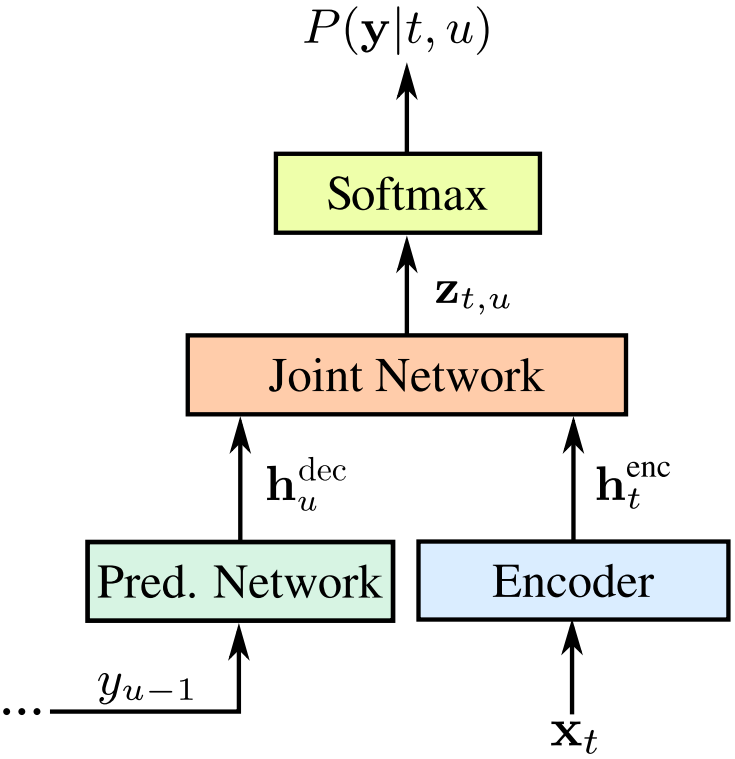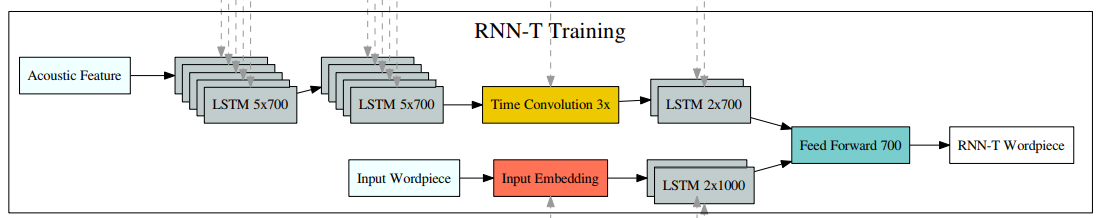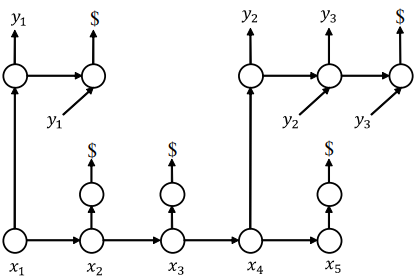# 【Learning Notes】Sequence Transducer

Sequence Transducer [1,2]，是 CTC 的一个扩展，也是由 Alex Graves 提出。相比于 CTC，Transducer 1）可以同时建模输入和输出的条件依赖（CTC 不依赖于输出）；2）对输入输出的长度没有限制（CTC 的输入长度不能小于输出）。这使得 Transducer 建模能力更加强大，理论上也可以解决更广泛的任务。

# 1. 模型概览# 2. 输入输出对齐

Transducer 采用了不同变长对齐处理方式：1）引入特殊 token Φ \varPhi （NULL，即空输出），类似 CTC的 blank；2）输出中的每个 token 只会出现一次。这样，输入输出的对齐反映在适当的位置出现 Φ \varPhi 。概念上，Transducer 的一个对齐如图3所示。# 3. 优化准则

CTC 中，由于输出概率独立，我们可以预计算出各个输入对应的输出概率分布，然后利用动态规划（前向后向算法）高效的计算似然及梯度，计算复杂度为 O ( T ⋅ U ) O(T\cdot U)

h t e n c = f e n c ( x t ) \mathbf{h}^{enc}_{t} = f^{enc}(x_{t})

h u d e c = f d e c ( y u − 1 ) \mathbf{h}^{dec}_{u} = f^{dec}(y_{u-1})

z t , u = f j o i n t ( h t e n c , h u d e c ) z_{t,u} = f^{joint}(\mathbf{h}^{enc}_{t},\mathbf{h}^{dec}_{u})

z t , u = M L P ( c a t ( h t e n c , h u d e c ) ) z_{t,u} = MLP(cat(\mathbf{h}^{enc}_{t}, \mathbf{h}^{dec}_{u}))

# 5. 相关代码

1. warp-rnnt.
2. warp-transducer （CPU/GPU）及 MXNet 绑定
3. C++ 实现及 tensorflow 绑定
4. C 实现及 pytorch 绑定，以及基于 python 的参考实现
5. 基于 RNN Transducer 的 音素识别（TIMIT） 示例

# References

02-242万+03-051万+
04-0666
03-148369
06-172295
11-204194
10-25192
09-241810
05-07482
05-07668
03-132万+
09-26515
04-283569
02-0342